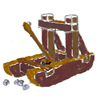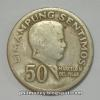#### You may also like### Oh for the Mathematics of Yesteryear

A garrison of 600 men has just enough bread ... but, with the news that the enemy was planning an attack... How many ounces of bread a day must each man in the garrison be allowed, to hold out 45 days against the siege of the enemy?### Thirty Nine, Seventy Five

We have exactly 100 coins. There are five different values of coins. We have decided to buy a piece of computer software for 39.75. We have the correct money, not a penny more, not a penny less! Can you discover what the five different types of coins are worth and how many of each we have saved?### History of Money

If you would like a new CD you would probably go into a shop and buy one using coins or notes. (You might need to do a bit of saving first!) However, this way of paying for the things you want did not always exist. Find out more ...

# Currency Exchange

##### Age 11 to 14 Short Challenge Level:

The difference between the amounts
Dan: 9$\times$20p = £1.80
Ann: 8$\times$50p = £4.00
Difference: £2.20
Half of £2.20 = £1.10 needs to change hands (from Ann to Dan)

£1.10 = 50p + 3$\times$30p   $\rightarrow$ not OK because Ann has no 20p s to give to Dan
£1.10 = 3$\times$50p $-$ 2$\times$20p   $\rightarrow$ 5 coins exchanged

Finding the total amount of money
In total, Ann and Dan have 180p + 400p = 580p, so they need to end up with 290p each. Therefore Dan needs to receive at least three 50p coins from Ann to have enough money. Then Ann has 250p, so she needs at least two 20p coins from Dan, at which point they have 290p each. Therefore, at least 5 coins must change hands.

Algebra
Suppose Dan gives Ann $x$ $20$p coins and Ann gives Dan $y$ $50$p coins. Then Dan has $(180-20x+50y)$p and Ann has $(400-50y+20x)$p.

We need $180-20x+50y=400-50y+20x$, which is the same as $10y-4x=22$, that is $5y-2x=11$. The solution to this equation which minimises $x+y$ is $x=2$, $y=3$, so the smallest number of coins that must change hands is $5$.

This problem is taken from the UKMT Mathematical Challenges.
You can find more short problems, arranged by curriculum topic, in our short problems collection.